# The Falkner Skan boundary layer

Here we have tried to validate the falkner skan code but we met some problems http://basilisk.fr/sandbox/easystab/falkner_skan.m

We use the analytical Pohlhausen equation to compare the final result but we didn’t ad the pressure gradient part of the equation. We use an equation for u0 of the form: \displaystyle u/ue = a + b eta +c eta^2 + d eta^3 + e eta ^4 were eta = y/d99

we use the boundary conditions to solv it: \displaystyle eta = 0 , u = 0 \displaystyle eta = 1 , u = ue \displaystyle eta = 1 , u_{y} = 0 \displaystyle eta = 1 , u_{yy} = 0

and \displaystyle (mu*u_{yy})|_{y=0} = -ue * ue_{x}

And by solving it we find the following equation:

\displaystyle u/ue = 2eta - 2eat^3 + eta^4 + lambda*eta*((1-eta)^3)/6

Were lambda is the parameter that introduce the pressure gradient factor. the last term isn’t introduced in the code and so the Pohlhausen equation we used can be compared only with the case where beta = 0.

clear all; clf; %setenv(“GNUTERM”,“X11”) % parameters L=12; % box height N=100; % number of gridpoints beta=-0.1; % pressure gradient parameter % differentiation matrices scale=-2/L; [y,DM] = chebdif(N,3); dy=DM(:,:,1)scale;
dyy=DM(:,:,2)
scale^2;
dyyy=DM(:,:,3)scale^3; y=(y-1)/scale;
I=eye(N); Z=zeros(N,N); % initial guess from order 4 Polhausen profile d99=6
sqrt(35/37); eta=y/d99; deta=dyd99; % rescaled vertical coordinate u0=1-(1-eta).^3.(1+eta); u0(eta>1)=1; A=deta; A(1,:)=I(1,:); u0(1)=0; % set up integration g0=A; % compute integral sol=g0 % Newton iterations quit=0;count=0; while ~quit % the present solution g=sol; gy=dyg; gyy=dyyg; gyyy=dyyy*g;

# The FS equation

Here is the nonlinear equation that we wish to solve \displaystyle 0=g_{yyy}+gg_{yy} + \beta (1 - g_y^2)

\displaystyle f(0) = f'(0), f'(infinite) = 1

The beta parameter introduce the pressure gradient. The infinite speed is of the form: \displaystyle ue = kx^{(beta/(2-beta))} The analytical Jacobian is \displaystyle A=\partial_{yyy}+g\partial_{yy}+g_{yy}I -2 \beta g_y \partial_{y}

    % nonlinear function
f=gyyy+g.*gyy+beta*(1-gy.^2);

% analytical jacobian
A=dyyy+diag(g)*dyy+diag(gyy)-2*beta*diag(gy)*dy;


# Boundary conditions

The boundary conditions are homogeneous Dirichlet and Neuman at the wall and Neuman 1 at the top. The constraint matrix for the boundary conditions is thus \displaystyle C=\left(\begin{array}{c} I|_0\\ \partial_y|_0\\ \partial_y|_L \end{array}\right) so that the homogeneous and nonhomogeneous boundary conditions are expressed \displaystyle Cg=\left(\begin{array}{c} 0\\ 0\\ 1 \end{array}\right)

    % Boundary conditions
loc=[1,2,N];
C=[I(1,:); dy(1,:); dy(N,:)];
f(loc)=C*g-[0;0;1];
A(loc,:)=C;

% convergence test
res=norm(f);
disp([num2str(count) '  ' num2str(res)]);
if count>50|res>1e5; disp('no convergence'); break; end
if res<1e-5; quit=1; disp('converged'); continue; end

% Newton step
sol=sol-A\f;
count=count+1;
end


To recover the velocity profile from g is \displaystyle u=g_y

% the solution
u=dy*g;

% Compute the displacement thickness and normalize it to unity
INT=([diff(y)',0]+[0,diff(y)'])/2; % integration weights
mt=INT*(1-u);
%%y=y/mt;

% Showing the result
plot(u,y,'b-',u0,y,'r--'); xlabel('u'); ylabel('y'); ylim([0,y(end)]);
title('Falkner-skan boundary layer');
legend('numerical','Pohlhausen');
grid on

set(gcf,'paperpositionmode','auto');
print('-dpng','-r80','falkner_skan.png');


# Result and Plot

We se in this figure the shape of à non zero beta for the falkner skan equation compared to our analytical Paulhausen that don’t inegrate the pressure gardient.The velocity profile

The problem is to find a relation between beta and lambda. We couldn’t solv this problem but we could study the shape and the behaviour of the falkner skan profile.

Here we ploted the simulation resul for différent values of beta: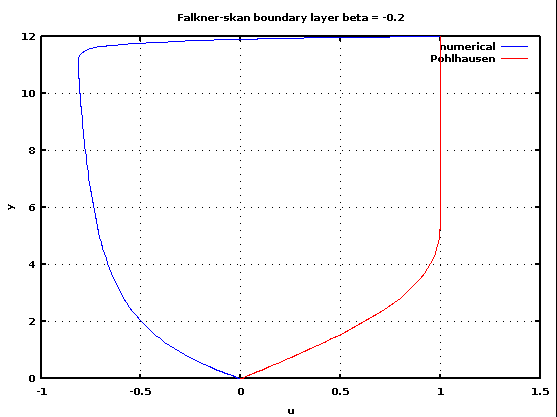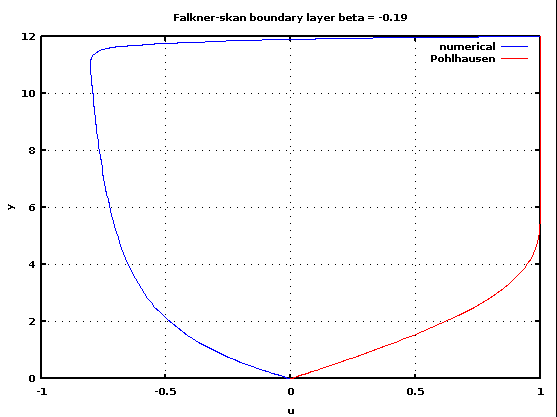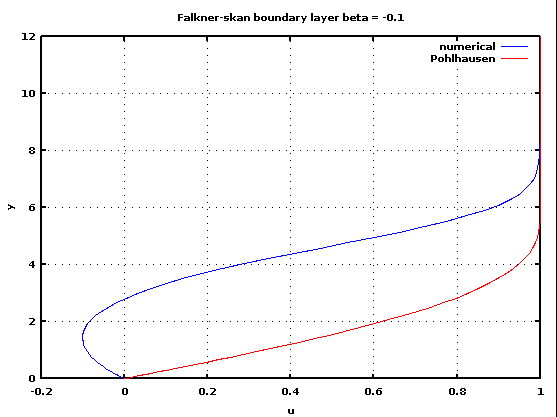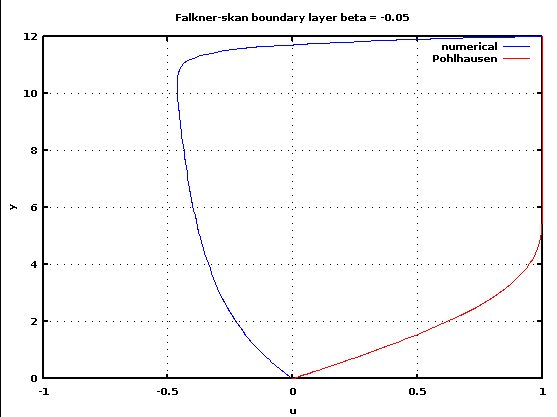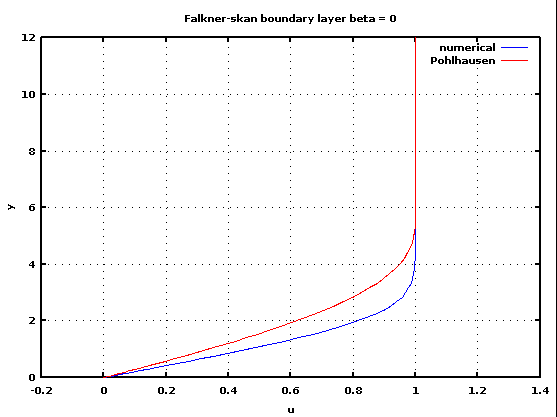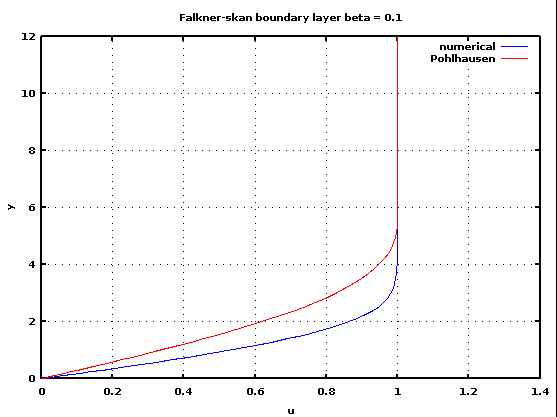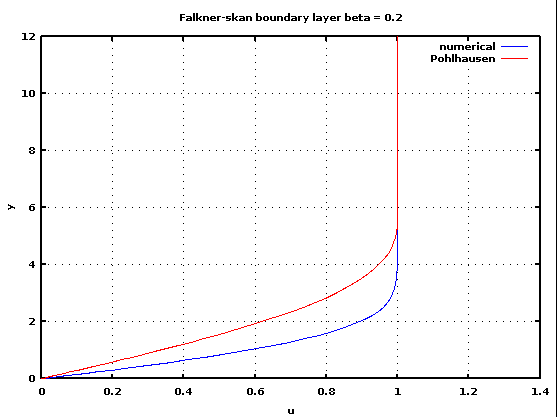Here we see à similar profile found in the internet: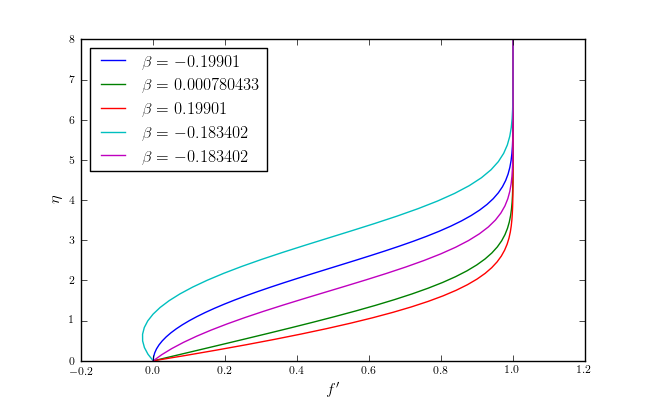Velocity profile from the web

We see that in the case of beta = -0.19 and beta = -0.05 the shape of the profile is not correct. We also notice that for beta = 0 the profile does not match with the Polhausen one. So we unvalidate that code and suggest to restudy it with deeper analysis to make it more accurate. The code were runned in the Octave software but we don’t think that could cause the unstability of the code.

When corrected the code should be validated and it can be done with values from other simulations found in the web if the sollution to link beta and lambda is not found.

# The sugestion answered to in Falkner_skan was:

Please validate this result with Pohlhausen profile.
Please validate the behavior for large β (which is the self similar flow in a convergent).